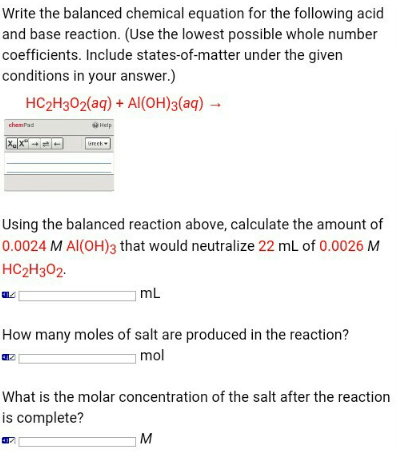# Problem: Write the balanced chemical equation for the following acid and base reaction. (Use the lowest possible whole number coefficients. Include states-of-matter under the given conditions in your answer.) HC2H3O2 (aq) + Al (OH)3 (aq) → Using the balanced reaction above, calculate the amount of 0.0024 M Al(OH) 3 that would neutralize 22 mL of 0.0026 M HC2H3O2.How many moles of salt are produced in the reaction? What is the molar concentration of the salt after the reaction is complete?

###### FREE Expert Solution
88% (226 ratings)###### Problem Details

Write the balanced chemical equation for the following acid and base reaction. (Use the lowest possible whole number coefficients. Include states-of-matter under the given conditions in your answer.)

HC2H3O(aq) + Al (OH)(aq) →

Using the balanced reaction above, calculate the amount of 0.0024 M Al(OH) 3 that would neutralize 22 mL of 0.0026 M HC2H3O2.

How many moles of salt are produced in the reaction?

What is the molar concentration of the salt after the reaction is complete?